# Mathematical Treatments and Early Models of Skin Permeability

(3.1)

where the terms used are shown in Fig. 3.1. This expression indicates that, at long times, there is a steady-state flux of (A 1 k 12 k 23)/(k 21 + k 23) and a lag time of 1/(k 21 + k 23), where A 1 k 12 = k p C v (Wallace and Barnett 1978; Roberts et al. 1999).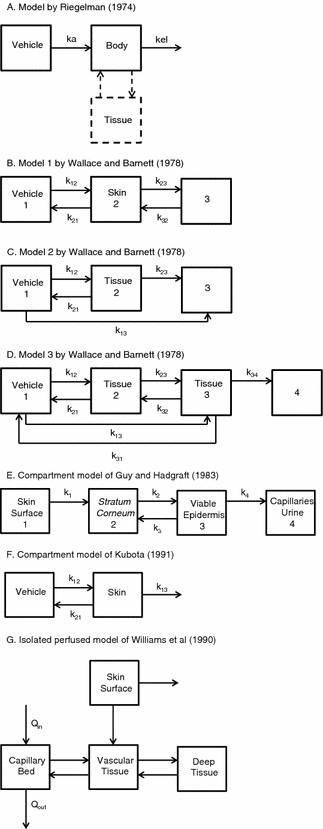Fig. 3.1
Examples of compartmental models of skin permeation (modified from Roberts et al. 1999)

Guy and Hadgraft (1982) used a pharmacokinetic model to describe in vivo blood and urine levels after topical application, and to relate this to the physical processes governing absorption. This is shown in Fig. 3.2 and illustrates the four-component pharmacokinetic model derived by Guy and Hadgraft (1982). It describes the process of drug diffusion through the stratum corneum, k 1, and the epidermis, k 2. Additionally, this model takes into account the reservoir effect of the stratum corneum by measuring the relative affinities of the penetrant for the stratum corneum and viable epidermis, represented by k 3. Finally, k 4 portrays the classical pharmacokinetic rate constant following intravenous administration. This model may be further modified by taking into account the vehicle effects, and as such may be used to screen potential transdermal formulation candidates. For example, it was applied to a study of the topical administration of sunscreens by Walters et al. (1998), and Guy et al. (1983) used it to estimate plasma levels resulting from multiple dosing of topically applied formulations. This model was modified by Guy and Hadgraft (1984) to include epidermal metabolism (notated by k 5 and k 6, which represented a metabolic step and the removal of the metabolite, respectively) by the inclusion of an additional compartment in the model.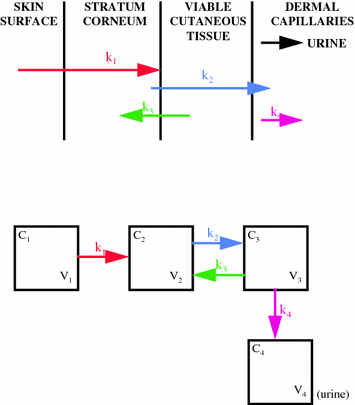Fig. 3.2
Fourcompartment pharmacokinetic model (modified from Guy and Hadgraft 1982)

In general, mathematical models relating to the specific penetration and absorption of a particular chemical may require a specific model due to the physicochemical properties of the penetrant and its specific pharmacokinetic and pharmacodynamic profile in the body. One example is the penetration of a local anaesthetic drug, which may be somewhat simplified as the site of action is at or near the dermo-epidermal junction. Thus, the resistance from the dermis may therefore be neglected, and systemic uptake is not relevant to the efficacy of such drugs. The total resistance of the skin, represented by R, to percutaneous absorption of a local anaesthetic can be described by:(3.2)
where

h

the thickness of the resistor membrane;

F

fractional area of the route, where there is more than one pathway involved;

D

diffusion coefficient of the drug;

K

partition coefficient of the drug;
and the denominator subscripts sc and e refer to the stratum corneum and the epidermis, respectively (Woolfson and McCafferty 1993).

It should also be noted that diffusion-based pharmacokinetic models, such as that described by Cooper (1976), have been applied to percutaneous absorption. Cooper assumed diffusion through the skin according to the flux of solutes exiting the membrane per unit area, J s(t), and used a single-compartment model to do so: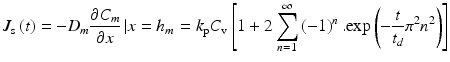(3.3)
where J s(t) is the flux of solutes exiting the membrane per unit area of the membrane.

Roberts et al. (1999) commented that the application of this model to in vivo absorption might be limited in describing percutaneous absorption as it is a single-compartment model. Rather, they suggest the following expression:(3.4)
Equation 3.4 was suggested as a more appropriate model as it takes into account the effectiveness of blood flow in removing the penetrant from the epidermis and the accumulation of the penetrant in vivo, and may be more appropriate to model the total process of percutaneous absorption (in the context of systemic absorption, i.e. transdermal drug delivery), as it considers drug absorption and elimination.

## Infinite and Finite Dosing

The concepts and experimental requirements for infinite and finite doses are discussed briefly in Chap. 2 and will also be discussed in greater detail in Chap. 8, where examples of finite-dose models will be discussed. They are also outlined below in the context of their underpinning mathematics.

An infinite-dose experiment is one where the concentration of permeant in the donor compartment remains constant and dose not therefore lessen over the duration of the experiment. Examples of infinite-dose experiments include those where saturated or supersaturated solutions, or transdermal patches, are applied to the skin surface. On the other hand, finite-dose experiments are those where a small amount of the penetrant of interest is applied to the skin surface and which will diminish during the course of the experiment. Examples of finite-dose experiments include those that consider the application of pharmaceutical creams and ointments or the application under non-occluded conditions formulations that include volatile components (normally solvents).

Transport of exogenous chemicals into and across the skin is characterised by its flux (J), that is, the concentration per unit area per unit time that passes across the skin. To date, no active transport mechanism has been identified for this process and it is understood to progress by passive diffusion due to a thermodynamic force based on a concentration gradient of the penetrant either side of the membrane across which diffusion occurs.

In the context of the predictive modelling of skin absorption, quantitative structure-activity (or permeability) relationships (QSARs or QSPRs) aim to relate statistically the biological activity of a range of penetrants (the data set from which the model is constructed) to the structural and physicochemical properties of penetrants and their experiments (Sinko 2010). More recently, as is described in Chap. 9, experimental parameters may additionally be added to models. Such processes result in models that provide two main functions: as they are normally in the form of an algorithm (i.e. an equation), they can offer mechanistic insight to the key structural and physicochemical parameters that influence the absorption process, and they can allow, by substitution of the data for a new chemical into the derived equation, a prediction of the permeability for that chemical. However, it may be the case that an algorithm featuring specific, discrete parameters suggests that such parameters do not exhibit any degree of overlap or covariance—as, for example, we see with molecular weight and melting point as we increase the carbon number of a series of n-alcohols. Therefore, the potentially composite or covariate nature of some descriptors employed in modelling studies should be considered when interpreting the output of models.

The aim of this book, and more specifically this chapter, is to consider the experimental and mathematical context in which quantitative structure–permeability relationships are derived. This chapter will focus on the mathematics underlying in vitro infinite-dose experiments, as the vast majority of these models are based on data sets constructed from experiments of that nature, where conditions of pseudo-steady-state permeation exist. While the system we are interested in is biological in nature, we are not examining a biological process, such as protein binding, binding to a receptor or active transport, but rather a passive diffusion process in a biological system. Nevertheless, as we have seen with some of the examples above, the overall diffusion process should be considered in the context of pharmacokinetics and metabolism, something which simple models/algorithms of permeability do not currently incorporate.

The models described above are extremely complex and relate to a range of circumstances, including finite and infinite doses and also consider the adsorption–distribution–metabolism–elimination (ADME) process in the context of percutaneous absorption. They have been described considerably in the excellent article by Roberts et al. (1999). However, in the context of algorithms for percutaneous absorption, a significant simplification of such models is often considered and relates to the application of principles of Fickian diffusion to the problem domain of percutaneous absorption.

This, Fick’s first law has been used to describe the transfer of a diffusing material through a membrane (Barry 1983):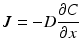(3.5)
where

J

is the flux of the permeant (the rate of transfer per unit area per unit time);

C

is the concentration of the diffusing substance;

x

is the spatial coordinate measured normal to the section (meaning that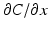is the concentration gradient across the membrane); and

D

is the diffusion coefficient, or the diffusivity of the penetrant.

According to Blank (1969), this model may only be applied to relatively low concentrations of penetrant, as deviations from Fick’s first law become apparent when the concentration increases greatly.

From Eq. 3.5, Fick’s second law of diffusion can be derived. It is an equation of mass transport, emphasising the change in concentration, not mass, per unit time diffusing across a barrier membrane. It assumes that penetration is unidirectional which, in an isotropic medium, may be given by:(3.6)
where t represents time.

Equation 3.6 may also be written in a more general form to represent three dimensions:(3.7)
However, most diffusional movement is in one direction only, and Eq. 3.5 adequately describes most experimental diffusional cases (Tojo 1987).

Fick’s second law states that “the change in concentration with time in a particular region is proportional to the change in the concentration gradient at that point in the system” (Martin et al. 1983). Solving Fick’s second law is, however, difficult and depends on boundary conditions for a particular experiment. For example, Franz (1975), using his eponymous diffusion cells and an infinite-dose experimental protocol, modified Fick’s equation and represented the amount of drug in the donor phase with the following expression: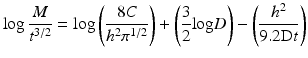(3.8)
where

M

cumulative amount of drug in receptor phase;

D

diffusion coefficient of drug in skin;

h

thickness of skin;

C

concentration of drug in donor compartment; and

t

time elapsed in the experiment.

However, while Eq. 3.8 has been successfully applied by other researchers (for example, Albery and Hadgraft 1979), simpler expressions are generally more frequently employed in both experimental and modelling experiments of percutaneous absorption.

Thus, following from the comments above, descriptions in Chap. 1 of the complex nature of the skin and, in Chap. 2, of the range of experiments (including the use of different mammalian skin tissues, or parts of skin tissue such as isolated stratum corneum or dermatomed skin, or even artificial membranes such as polydimethylsiloxane) used for particular experiments, it follows that the application of Fick’s law to diffusion offers, at best, an approximation of the diffusion process across the skin, a comment which should be considered in the wider context of how closely in vitro experiments can mimic in vivo conditions and—in the wider context of this book—how accurate models of absorption can be if they, as their theoretical basis, use models based on simplifications and approximations. As was highlighted in Chap. 2, the manner in which this is achieved usually relates to an experiment where the membrane of interest (i.e. whole skin, isolated stratum corneum, epidermis, dermatomed skin or an artificial membrane) sits between two compartments: the donor compartment from where the chemical of interest will diffuse and the receptor compartment into which it will diffuse. After a period of equilibration (often referred to as the “lag phase” of the diffusion process) steady-state diffusion will be achieved when the concentration gradient across the membrane is constant, in which case Fick’s second law (Eq. 3.6) may be written as:(3.9)
where

M

is the cumulative mass of permeant passing across the membrane per unit area per unit time (t);

C 0

is the concentration of the permeant at the skin surface; and

h

is the thickness of the membrane.

C 0 is, in practice, difficult to measure, and the concentration in the donor solution or formulation, C V, is normally used as a readily measurable approximation. C 0 and C V are related by P, the partition coefficient of the permeant between the vehicle and the membrane:(3.10)
and(3.11)

Substitution of Eq. 3.8 into Eq. 3.6 gives the following expression: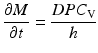(3.12)
Thus, a plot of M (the cumulative amount of permeant, passing across a defined area of the membrane (as for example, μg/cm2 against time)) results in a profile of permeation, from the donor compartment, across the membrane and into the receptor compartment. The lag time can be determined by extrapolating the pseudo-steady-state area of the diffusion profile to the x(time)-axis, or after the method derived by Crank (1975):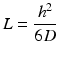(3.13)
where L is the lag time.

This equation infers that the diffusion coefficient can be determined directly from the lag time. However, while this holds for simple isotropic membranes, it does not apply to more complex membranes, such as mammalian skin. Further, Williams (2003) also commented that lag times obtained from experiments are highly variable and may be influenced by permeant-skin binding. An alternative approach is to rearrange Eq. 3.9:(3.14)

Given that is the gradient of the diffusion profile—the flux (J)—this expression may be simplified further:(3.15)
Equation 3.15 allows the calculation of the diffusion coefficient and, while still influenced by experimental variation, it is lessened as this expression does not contain squared terms, as Eq. 3.10 does, which comparatively reduces any error.

The main focus of QSPRs for skin permeability has not been the flux, but the permeability coefficient, k p. The permeability coefficient is the steady-state flux normalised by the concentration gradient across the membrane. This may be expressed by:(3.16)
where

J ss

is the steady-state flux determined from the diffusion profile (with units of concentration per unit area per unit time);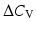is the concentration gradient across the membrane.

The concentration gradient is maximised if the concentration below the membrane is essentially zero—in practice, this means maintaining sink conditions where possible. It also indicates, as discussed in Chap. 2 in the context of permeability experiments, how an alteration in the concentration gradient (by, for example, failing to maintain sink conditions or in the case of solvent loss in some finite-dose experiments) can influence the rate of diffusion and the flux across the skin. This is particularly important in the practical sense of maximising permeation across the skin of, for example, pharmaceutically active materials. Further, Crank (1975), in defining the skin as a single pseudo-homogenous membrane, suggested that Eqs. 3.14 and 3.16 may be rearranged to provide the following expression: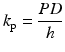(3.17)

Substituting into Eq. 3.12 this gives the following expression: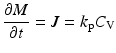(3.18)

The flux may be obtained from the gradient of the diffusion profile, being the gradient of the steady-state component of the concentration–time plot of permeant diffusion. k p may then be determined as the concentration gradient is known or can be approximated based on skin conditions. It should be noted that k p and C v should be determined in the same solvent (or vehicle) and that models cannot be developed using mixtures of solvents (i.e. with k p determined for an aqueous solvent and C v for a non-aqueous solvent). The permeability coefficient is often used to characterise skin permeability in preference to other parameters as is it relatively straightforward to determine and, as it normalises for concentration, allows comparisons of permeability for a range of permeants to be made. However, it is clear that J max is a more practical and useful term to use, particularly from pharmaceutical or toxicological perspectives, being used, for example, as a key characteristic of the clinical performance of a transdermal drug delivery device. Thus, in terms of QSPR models, skin absorption, as k p, is the most widely used term, although some significant studies, most notably Magnusson et al. (2004), derive models based on steady-state flux. As described in Chap. 2, it is a limitation of these models that they seldom account for formulation effects. However, k p is used as, over time, a large body of data has been published in this format, which allows comparisons of theoretical models with physical (i.e. experimental or clinical) measurements, and Kroes et al. (2007) have considered the use of J max and k p in the context of risk assessment for cosmetic formulations.